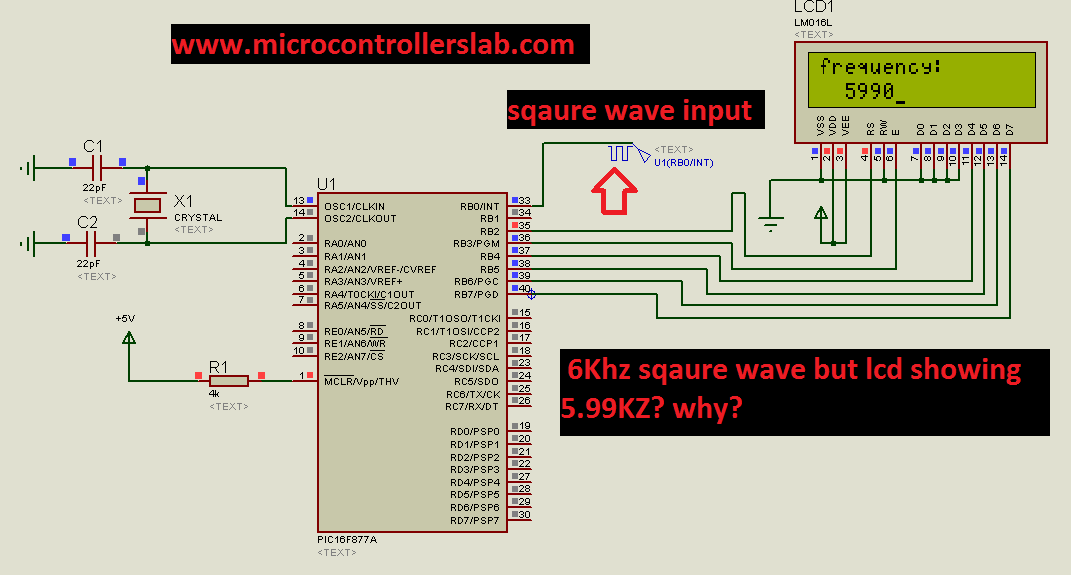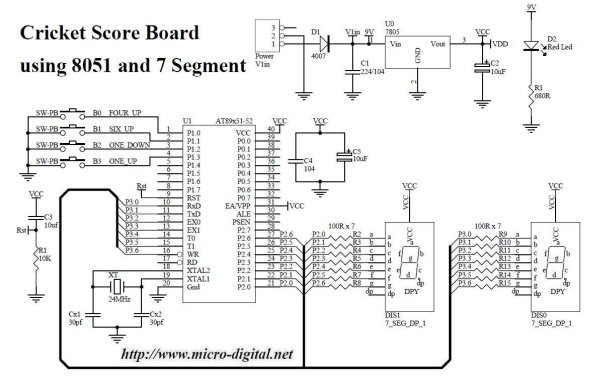# Digital frequency meter using pic microcontroller

Digital frequency meter with LCD display  can be used to measure frequency of square wave. With little bit modification in this embedded systems project you can also measure frequency of sine wave. I will also discuss it at the end of this article.In this digital frequency meter PIC16F877A microcontroller is used to measure frequency with the help of external interrupt and 16 * 2 LCD is used for digital display of frequency.#### Applications of Digital frequency meter

Digital frequency meter have many application. There are many sensors which don’t give output in voltage form. To interface these kind of sensors with digital system signal conditioning has to be done. For example in capacitive sensors extra circuit is used to convert change in capacitance to another physical parameter which a digital system can easily read.In capacitive sensors change in frequency with respect input is usually convert into proportional frequency square wave with the help of some extra circuitry. Example of such sensor is humidity sensor.

There are many other examples of digital frequency meter like :

# Digital frequency meter using pic microcontroller

#### Digital frequency meter with LCD display  using PIC16F877A

Digital frequency meter with LCD display  can be used to measure frequency of square wave. With little bit modification in this embedded systems project you can also measure frequency of sine wave. I will also discuss it at the end of this article.In this digital frequency meter PIC16F877A microcontroller is used to measure frequency with the help of external interrupt and 16 * 2 LCD is used for digital display of frequency.

#### Applications of Digital frequency meter

Digital frequency meter have many application. There are many sensors which don’t give output in voltage form. To interface these kind of sensors with digital system signal conditioning has to be done. For example in capacitive sensors extra circuit is used to convert change in capacitance to another physical parameter which a digital system can easily read.In capacitive sensors change in frequency with respect input is usually convert into proportional frequency square wave with the help of some extra circuitry. Example of such sensor is humidity sensor.

There are many other examples of digital frequency meter like :

#### Components

Complete list of components you need to make a digital frequency meter:

```Resistors,"R1",4k,
Capacitors,"C1",22pF,
Capacitors,"C2",22pF,
Integrated Circuits,"U1",PIC16F877A,
LCD,"LCD1",LM016L,
CRYSTAL,"X1",CRYSTAL 20Mhz,
```

#### Implementation

Frequency and time period are inversely proportional with each other .

Frequency = 1 / time period

If one know time period of one wave , frequency can also be easily calculated with above relation . we can’t measure frequency directly with this method. In this project time period of square wave is measured first which is converted into frequency by using above relationship of frequency and time period.

PIC16F8877A microcontroller externnal interrupt at rising edge and timer are used to measure time period of square wave. algorithm is developed to convert time period into frequency. 16 * 2 LCD is used to display measured frequency digitally.

In above circuit diagram pulse generator is used to generate square wave of any frequency.This is just for simulation purpose. You can connect any device to this pin which frequency you want to measure.We have connected 6Khz square wave with interrupt pin to measure its frequency. But LCD is showing 5.99 KHz. This is because of real time operating issues of microcontroller. I will not discuss them here. But to remove this error. Read frequency 5-10 times and display their average on LCD.

READ  PIC-based Digital Voltmeter (DVM)

For more detail: Digital frequency meter using pic microcontroller

#### Current Project / Post can also be found using:

• digital frequency meter in embedded system
• download digital frequency meter with pic16f877a by micro c
• frequency measurement using pic microcontroller
• phase changer using pic16f877